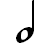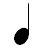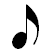The bottom number of a time signature tells us the type of rhythmic note that receives one beat of time. Let’s see how changing the bottom number changes the number of beats that each rhythmic note is worth.

Remember, the relationship between the rhythmic notes (twice as long, half as long, etc.) never changes. If you know which note gets one beat, then you can calculate the remaining note values based on their relationship to the beat note.

## Instructions

The columns on the left show the bottom number of a time signature.

For each note in that column, select the value that matches the number of beats that the note receives when the column number is the bottom number of a time signature.

For example, if the number is 4, select the number of beats that each note receives when a quarter note gets one beat (i.e., 4 is the bottom number of the time signature).

Bottom Number Time Signature How Many Beats is this Note Worth?
1 2Bottom Number Time Signature How Many Beats is this Note Worth?
4 8